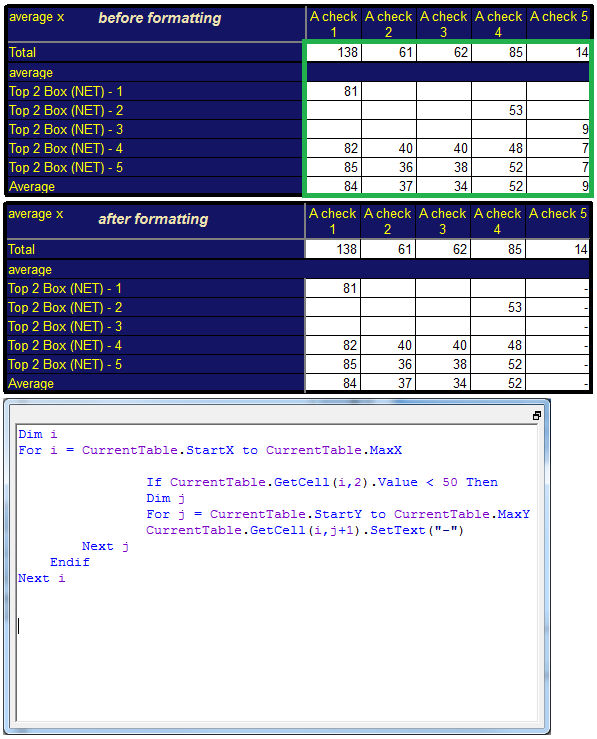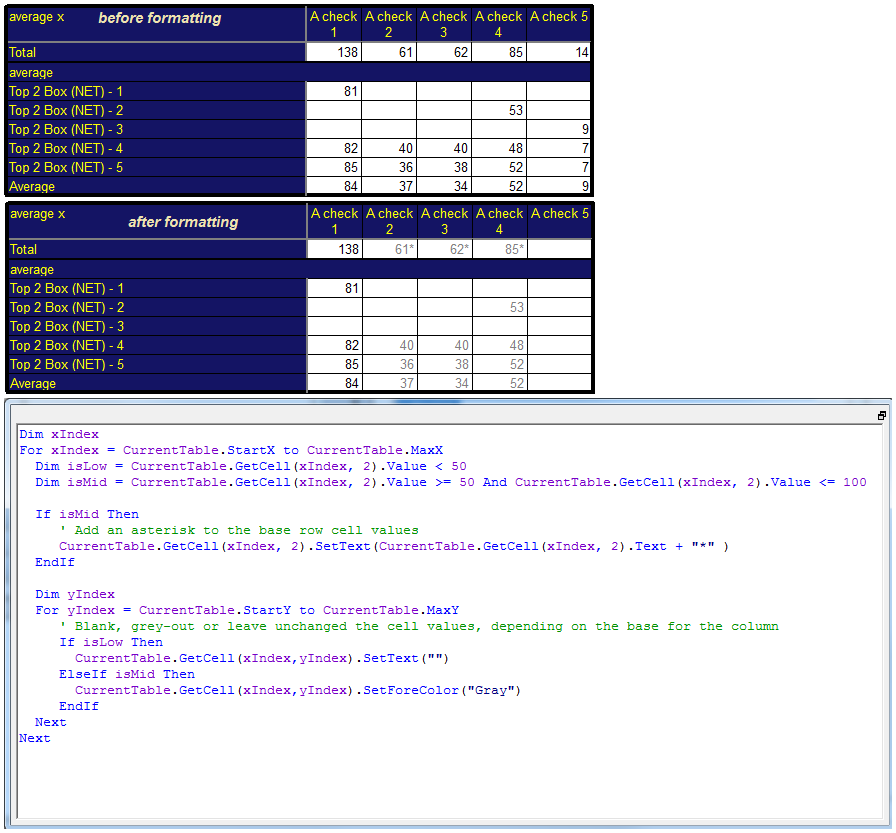Scroll

# Table Formatting

Follow
 Summary This article is the first in a series of articles that will go through examples of using table arithmetic syntax new to Askia 5.3.5.0. This article will demonstrate the post processing or 'Cleaning script' that changes the appearance of a table based on the figures it has in it. Applies to Askia Analyse. Written for Researchers, Developers, Data Processors. Keywords Post processing, Cleaning script, Format, Layout, Colour, Conditional formatting, Table arithmetic, Forloops, Coordinates, data.

The .qes file and portfolio containing both of the described examples is attached: Table Arithmetic Examples 1.rar.

1. Formatting script (Simple)

The requirement is to replace all entries in a column with ‘-‘ if the base row is less than 50.

You will need to apply script here: General tab > Settings > Sorting > Cleaning scriptCurrentTable.StartX is the X coordinate of the first column (left to right) where data is found

CurrentTable.StartY is the Y coordinate of the first row (top to bottom) where data is found

So the start of the data range, (X,Y), is (2,2), the cell in the top left of the data range reading 138.

CurrentTable.MaxX is the X coordinate of the last column (left to right) where data is found

CurrentTable.MaxY is the Y coordinate of the last row (top to bottom) where data is found

So the end of the data range, (X,Y), is (6,9), the cell in the bottom right of the data range reading 9.

1. Formatting script (Intermediate)

The requirement is to:

• Blank out all data (including base row) in any column where the base is less than 50
• For any column where the base is between 50 and 100, we need to:
• Change the text colour in the column to be grey
• Append an asterisk to the base count

You will need to apply script here: General tab > Settings > Sorting > Cleaning script